Q-Chem 5.1 User’s Manual

# 11.5 Visualizing and Plotting Orbitals, Densities, and Other Volumetric Data

The free, open-source visualization program IQmol (www.iqmol.org) provides a graphical user interface for Q-Chem that can be used as a molecular structure builder, as a tool for local or remote submission of Q-Chem jobs, and as a visualization tool for densities and molecular orbitals. Alternatively, Q-Chem can generate orbital and density data in formats suitable for plotting with various third-party visualization programs.

## 11.5.1 Visualizing Orbitals Using MolDen and MacMolPlt

Upon request, Q-Chem will generate an input file for MolDen, a freely-available molecular visualization program. MolDen can be used to view ball-and-stick molecular models (including stepwise visualization of a geometry optimization), molecular orbitals, vibrational normal modes, and vibrational spectra. MolDen also contains a powerful Z-matrix editor. In conjunction with Q-Chem, orbital visualization via MolDen is currently supported for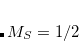,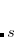, and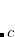functions (pure or Cartesian), as well as pure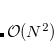functions. Upon setting MOLDEN_FORMAT to TRUE, Q-Chem will append a MolDen-formatted input file to the end of the Q-Chem log file. As some versions of MolDen have difficulty parsing the Q-Chem log file itself, we recommend that the user cut and paste the MolDen-formatted part of the Q-Chem log file into a separate file to be read by MolDen.

MOLDEN_FORMAT
 Requests a MolDen-formatted input file containing information from a Q-Chem job.

TYPE:
 LOGICAL

DEFAULT:
 FALSE

OPTIONS:
 TRUE Append MolDen input file at the end of the Q-Chem output file.

RECOMMENDATION:
 None.

MolDen-formatted files can also be read by MacMolPlt, another freely-available visualization program. MacMolPlt generates orbital iso-contour surfaces much more rapidly than MolDen, however, within MacMolPlt these surfaces are only available for Cartesian Gaussian basis functions, i.e., PURECART = 2222, which may not be the default.

Example 11.242  Generating a MolDen file for molecular orbital visualization.

$molecule 0 1 O H 1 0.95 H 1 0.95 2 104.5$end

$rem METHOD hf BASIS cc-pvtz PRINT_ORBITALS true (default is to print 5 virtual orbitals) MOLDEN_FORMAT true$end


For geometry optimizations and vibrational frequency calculations, one need only set MOLDEN_FORMAT to TRUE, and the relevant geometry or normal mode information will automatically appear in the MolDen section of the Q-Chem log file.

Example 11.243  Generating a MolDen file to step through a geometry optimization.

$molecule 0 1 O H 1 0.95 H 1 0.95 2 104.5$end

$rem JOBTYPE opt METHOD hf BASIS 6-31G* MOLDEN_FORMAT true$end


## 11.5.2 Visualization of Natural Transition Orbitals

For excited states calculated using the CIS, RPA, TDDFT, EOM-CC, and ADC methods, construction of Natural Transition Orbitals (NTOs), as described in Sections 7.12.2 and 11.2.6, is requested using the $rem variable NTO_PAIRS. This variable also determines the number of hole/particle NTO pairs that are output for each excited state and the number of natural orbitals or natural difference orbitlas. Although the total number of hole/particle pairs is equal to the number of occupied MOs, typically only a very small number of these pairs (often just one pair) have significant amplitudes. (Additional large-amplitude NTOs may be encountered in cases of strong electronic coupling between multiple chromophores.) NTO_PAIRS  Controls the writing of hole/particle NTO pairs for excited state. TYPE:  INTEGER DEFAULT:  0 OPTIONS: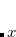WriteNTO pairs per excited state. RECOMMENDATION:  If activated (), a minimum of two NTO pairs will be printed for each state. Increase the value ofif additional NTOs are desired. When NTO_PAIRS, Q-Chem will generate the NTOs in MolDen format. The NTOs are state-specific, in the sense that each excited state has its own NTOs, and therefore a separate MolDen file is required for each excited state. These files are written to the job’s scratch directory, in a sub-directory called NTOs, so to obtain the NTOs the scratch directory must be saved using the –save option that is described in Section 2.7. The output files in the NTOs directory have an obvious file-naming convention. The “hole” NTOs (which are linear combinations of the occupied MOs) are printed to the MolDen files in order of increasing importance, with the corresponding excitation amplitudes replacing the canonical MO eigenvalues. (This is a formatting convention only; the excitation amplitudes are unrelated to the MO eigenvalues.) Following the holes are the “particle” NTOs, which represent the excited electron and are linear combinations of the virtual MOs. These are written in order of decreasing amplitude. To aid in distinguishing the two sets within the MolDen files, the amplitudes of the holes are listed with negative signs, while the corresponding NTO for the excited electron has the same amplitude with a positive sign. Due to the manner in which the NTOs are constructed (see Section 7.12.2), NTO analysis is available only when the number of virtual orbitals exceeds the number of occupied orbitals, which may not be the case for minimal basis sets. Example 11.244 Generating MolDen-formatted natural transition orbitals for several excited states of uracil. $molecule
0 1
N    -2.181263     0.068208     0.000000
C    -2.927088    -1.059037     0.000000
N    -4.320029    -0.911094     0.000000
C    -4.926706     0.301204     0.000000
C    -4.185901     1.435062     0.000000
C    -2.754591     1.274555     0.000000
N    -1.954845     2.338369     0.000000
H    -0.923072     2.224557     0.000000
H    -2.343008     3.268581     0.000000
H    -4.649401     2.414197     0.000000
H    -6.012020     0.301371     0.000000
H    -4.855603    -1.768832     0.000000
O    -2.458932    -2.200499     0.000000
$end$rem
METHOD          B3LYP
BASIS           6-31+G*
CIS_N_ROOTS     3
NTO_PAIRS       2
$end  ## 11.5.3 Generation of Volumetric Data Using$plots

The simplest way to visualize the charge densities and molecular orbitals that Q-Chem evaluates is via a graphical user interface, such as those described in the preceding section. An alternative procedure, which is often useful for generating high-quality images for publication, is to evaluate certain densities and orbitals on a user-specified grid of points. This is accomplished by invoking the $plots option, which is itself enabled by requesting IANLTY = 200. The format of the$plots input is documented below. It permits plotting of molecular orbitals, the SCF ground-state density, and excited-state densities obtained from CIS, RPA or TDDFT/TDA, or TDDFT calculations. Also in connection with excited states, either transition densities, attachment/detachment densities, or natural transition orbitals (at the same levels of theory given above) can be plotted as well.

By default, the output from the $plots command is one (or several) ASCII files in the working directory, named plot.mo, etc.. The results then must be visualized with a third-party program capable of making 3-D plots. (Some suggestions are given in Section 11.5.4.) An example of the use of the$plots option is the following input deck:

Example 11.245  A job that evaluates the H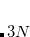HOMO and LUMO on agrid, along the bond axis. The plotting output is in an ASCII file called plot.mo, which lists for each grid point,,,, and the value of each requested MO.

$molecule 0 1 H 0.0 0.0 0.35 H 0.0 0.0 -0.35$end

$rem METHOD hf BASIS 6-31g** IANLTY 200$end

$plots Plot the HOMO and the LUMO on a line 1 0.0 0.0 1 0.0 0.0 15 -3.0 3.0 2 0 0 0 1 2$end


General format for the $plots section of the Q-Chem input deck.$plots
One comment line
Specification of the 3-D mesh of points on 3 lines:A line with 4 integers indicating how many things to plot:An optional line with the integer list of MO’s to evaluate (only if)
MO(1)  MO(2)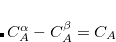MO()
An optional line with the integer list of densities to evaluate (only if)
Rho(1)  Rho(2)Rho()
An optional line with the integer list of transition densities (only if)
Trans(1)  Trans(2)Trans()
An optional line with states for detachment/attachment densities (if)
DA(1)  DA(2)DA()
$end Line 1 of the$plots keyword section is reserved for comments. Lines 2–4 list the number of one dimension points and the range of the grid (note that coordinate ranges are in Ångstroms, while all output is in atomic units). Line 5 must contain 4 non-negative integers indicating the number of: molecular orbitals (), electron densities (), transition densities and attach/detach densities (), to have mesh values calculated.

The final lines specify which MOs, electron densities, transition densities and CIS attach/detach states are to be plotted (the line can be left blank, or removed, if the number of items to plot is zero). Molecular orbitals are numbered; electron densities numbered where 0= ground state, 1 = first excited state, 2 = second excited state, etc.; and attach/detach specified from state.

By default, all output data are printed to files in the working directory, overwriting any existing file of the same name.

• Molecular orbital data is printed to a file called plot.mo;

• Densities are plotted to plots.hf;

• Restricted unrelaxed attachment/detachment analysis is sent to plot.attach.alpha and
plot.detach.alpha;

• Unrestricted unrelaxed attachment/detachment analysis is sent to plot.attach.alpha,
plot.detach.alpha, plot.attach.beta and plot.detach.beta;

• Restricted relaxed attachments/detachment analysis is plotted in plot.attach.rlx.alpha and
plot.detach.rlx.alpha; and finally

• Unrestricted relaxed attachment/detachment analysis is sent to plot.attach.rlx.alpha,
plot.detach.rlx.alpha, plot.attach.rlx.beta and plot.detach.rlx.beta.

Output is printed in atomic units, with coordinates first followed by item value, as shown below:

x1   y1   z1      a1   a2  ...  aN
x2   y1   z1      b1   b2  ...  bN
...


Instead of a standard one-, two-, or three-dimensional Cartesian grid, a user may wish to plot orbitals or densities on a set of grid points of his or her choosing. Such points are specified using a $grid input section whose format is simply the Cartesian coordinates of all user-specified grid points: x1 y1 z1 x2 y2 z2 ...  The$plots section must still be specified as described above, but if the $grid input section is present, then these user-specified grid points will override the ones specified in the$plots section.

The Q-Chem $plots utility allows the user to plot transition densities and detachment/attachment densities directly from amplitudes saved from a previous calculation, without having to solve the post-SCF (CIS, RPA, TDA, or TDDFT) equations again. To take advantage of this feature, the same Q-Chem scratch directory must be used, and the SKIP_CIS_RPA$rem variable must be set to TRUE. To further reduce computational time, the SCF_GUESS $rem can be set to READ. SKIP_CIS_RPA  Skips the solution of the CIS, RPA, TDA or TDDFT equations for wave function analysis. TYPE:  LOGICAL DEFAULT:  FALSE OPTIONS:  TRUE / FALSE RECOMMENDATION:  Set to true to speed up the generation of plot data if the same calculation has been run previously with the scratch files saved. ### 11.5.3.1 New$plots input

New format for the $plots section provides readable and friendly input for generation of volumetric data. The input section can be divided into three parts. The first part contains basic plot options which define the 3-D mesh of points. The second part contains the selection of densities or orbitals. The advanced options are included in the last part. With new plot format, there are multiple ways to define 3-D mesh points. If no plot option is given, the boundaries of the mesh box are the maximum/minimum molecular coordinatesÅ. The default box can be simply enlarged or reduced by setting grid_range to a value larger or smaller than(negative number is accepted), respectively. To customize the mesh box, set grid_range to desired boundaries: $plots
grid_range (-1,1)  (-1,1)  (-1,1)
$end  This defines a 2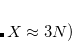22 mesh box centered at the molecular coordinate origin. Note that there is no space in the parentheses. The number of one dimension points is the value of the box length divided by grid_spacing. The default grid point spacing isÅ. To override the usage of grid_spacing and customize the number of 3-D points, set grid_points to desired values. To generate cube file (Section 11.5.4) using new plot format, just set MAKE_CUBE_FILES to TRUE in$rem section. The new plot format is enabled by requesting PLOTS = 1.

 Option Explanation Basic plot options grid_range boundaries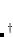of the mesh box or increment/decrement in the default boundariesin Å grid_spacing grid point spacingin Å grid_points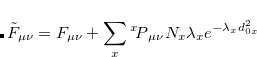Density/orbital selectionalpha_molecular_orbital a integer range of alpha MO’s to evaluate beta_molecular_orbital a integer range of beta MO’s to evaluate total_density a integer range of total densities to evaluate spin_density a integer range of spin densities to evaluate transition_density a integer range of transition densities to evaluate attachment_detachment_density a integer range of det.-att. densities to evaluate Advanced options reduced_density_gradient invoke non-covalent interaction (NCI) plot orbital_laplacians evaluate orbital Laplacians average_local_ionization evaluate average local ionization energies with a given contour value of the electron density. The default is().the format:the default isÅ increment in the boundaries derived from the molecular coordinatesthe default isÅ; it can be overridden by option ’grid_points’input format: n-m or n, indicating n-th oribtal or state; use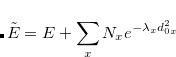for the ground-state
Table 11.3: Options for new $plots input section Example 11.246 Generating the cube files: the total densities of the ground and the first two excited states, the transition and detachment/attachment densities of the first two excited states, and the 28th to 31th alpha molecular orbitals, with customized 3-D mesh box and points. $rem
method          cis
basis           6-31+G*
cis_n_roots     4
cis_triplets    false
make_cube_files true   ! triggers writing of cube files
plots           true
$end$plots
grid_range  (-8,8) (-8,8) (-8,8)
grid_points 40 40 40
total_density 0-2
transition_density 1-2
attachment_detachment_density 1-2
alpha_molecular_orbital   28-31
$end$molecule
0 1
C         -4.57074        2.50214       -0.00000
O         -3.35678        2.38653        0.00000
H         -5.18272        1.79525       -0.54930
H         -5.03828        3.31185        0.54930
$end  Example 11.247 Generating the cube files of the average local ionization energies and the total density for the ground state of aniline. $rem
jobtype = sp
exchange = hf
basis = 6-31g*
make_cube_files = true
plots = true
$end$plots
grid_spacing  0.1
total_density 0
average_local_ionization
$end$molecule
0 1
H        -2.9527248536   -0.0267579494    0.0000000000
C        -1.8714921071   -0.0106828899    0.0000000000
C        -1.1721238067   -0.0011274864   -1.1972702914
H        -1.7092440589   -0.0094706668   -2.1378190515
C         0.2115215040    0.0174872124   -1.2026764462
H         0.7547333507    0.0243282741   -2.1379453065
C         0.9165181187    0.0252342217    0.0000000000
N         2.3578744287    0.1198186854    0.0000000000
H         2.7471831094   -0.3464272024   -0.8299201716
H         2.7471831094   -0.3464272024    0.8299201716
C         0.2115215040    0.0174872124    1.2026764462
H         0.7547333507    0.0243282741    2.1379453065
C        -1.1721238067   -0.0011274864    1.1972702914
H        -1.7092440589   -0.0094706668    2.1378190515
$end  ## 11.5.4 Direct Generation of “Cube” Files As an alternative to the output format discussed above, all of the$plots data may be output directly to a sub-directory named plots in the job’s scratch directory, which must therefore be saved using the –save option described in Section 2.7. The plotting data in this sub-directory are not written in the plot.* format described above, but rather in the form of so-called “cube” file, one for each orbital or density that is requested. The cube file format is a standard one for volumetric data and consists of a small header followed by the orbital or density values at each grid point, in ASCII format. (Consult Ref. Herbert:2015 for the complete format specification.) Because the grid coordinates themselves are not printed (their locations are implicit from information contained in the header), each individual cube file is much smaller than the corresponding plot.* file would be. Cube files can be read by many standard (and freely-available) graphics programs, including MacMolPlt and VMD. VMD, in particular, is recommended for generation of high-quality images for publication. Cube files for the MOs and densities requested in the $plots section are requested by setting MAKE_CUBE_FILES to TRUE, with the$plots section specified as described in Section 11.5.3.

MAKE_CUBE_FILES
 Requests generation of cube files for MOs, NTOs, or NBOs.

TYPE:
 LOGICAL/STRING

DEFAULT:
 FALSE

OPTIONS:
 FALSE Do not generate cube files. TRUE Generate cube files for MOs and densities. NTOS Generate cube files for NTOs. NBOS Generate cube files for NBOs.

RECOMMENDATION:
 None

PLOT_SPIN_DENSITY
 Requests the generation of spin densities,and.

TYPE:
 LOGICAL

DEFAULT:
 FALSE

OPTIONS:
 FALSE Do not generate spin density cube files. TRUE Generate spin density cube files.

RECOMMENDATION:
 Set to TRUE if spin densities are desired in addition to total densities. Requires that MAKE_CUBE_FILES be set to TRUE as well, and that one or more total densities is requested in the $plots input section. The corresponding spin densities will then be generated also. The following example illustrates the generation of cube files for a ground and an excited-state density, including the corresponding spin densities. In this example, the plots sub-directory of the job’s scratch directory should contain files named dens.N.cube (total density for state, whereor 1 represents the ground and first excited state, respectively), dens_alpha.N.cube and dens_beta.N.cube (andfor each state), and dens_spin.N.cube (for each state.) Example 11.248 Generating density and spin-density cube files for the ground and first excited state of the HOO radical. $molecule
0 2
H     1.004123   -0.180454    0.000000
O    -0.246002    0.596152    0.000000
O    -1.312366   -0.230256    0.000000
$end$rem
PLOT_SPIN_DENSITY  true
MAKE_CUBE_FILES    true
SCF_CONVERGENCE    8
METHOD             b3lyp
BASIS              6-31+G*
CIS_N_ROOTS        1
$end$plots
grid information and request to plot 2 densities
20  -5.0  5.0
20  -5.0  5.0
20  -5.0  5.0
0  2  0  0
0  1
$end  Cube files are also available for natural transition orbitals (Sections 7.12.2 and 11.5.2) by setting MAKE_CUBE_FILES to NTOS, although in this case the procedure is somewhat more complicated, due to the state-specific nature of these quantities. Cube files for the NTOs are generated only for a single excited state, whose identity is specified using CUBEFILE_STATE. Cube files for additional states are readily obtained using a sequence of Q-Chem jobs, in which the second (and subsequent) jobs read in the converged ground- and excited-state information using SCF_GUESS and SKIP_CIS_RPA. CUBEFILE_STATE  Determines which excited state is used to generate cube files TYPE:  INTEGER DEFAULT:  None OPTIONS: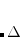Generate cube files for theth excited state RECOMMENDATION:  None An additional complication is the manner in which to specify which NTOs will be output as cube files. When MAKE_CUBE_FILES is set to TRUE, this is specified in the$plots section, in the same way that MOs would be specified for plotting. However, one must understand the order in which the NTOs are stored. For a system with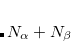-spin MOs, the occupied NTOsare stored in order of increasing amplitudes, so that the’th occupied NTO is the most important. The virtual NTOs are stored next, in order of decreasing importance. According to this convention, the highest occupied NTO (HONTO)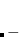lowest unoccupied NTO (LUNTO) excitation amplitude is always the most significant, for any particular excited state. Thus, orbitalsandrepresent the most important NTO pair, while orbitalsandrepresent the second most important NTO pair, etc..

Example 11.249  Generating cube files for the HONTO-to-LUNTO excitation of the second singlet excited state of uracil. Note thatfor uracil.

$molecule 0 1 N -2.181263 0.068208 0.000000 C -2.927088 -1.059037 0.000000 N -4.320029 -0.911094 0.000000 C -4.926706 0.301204 0.000000 C -4.185901 1.435062 0.000000 C -2.754591 1.274555 0.000000 N -1.954845 2.338369 0.000000 H -0.923072 2.224557 0.000000 H -2.343008 3.268581 0.000000 H -4.649401 2.414197 0.000000 H -6.012020 0.301371 0.000000 H -4.855603 -1.768832 0.000000 O -2.458932 -2.200499 0.000000$end

$plots Plot the dominant NTO pair, N --> N+1 25 -5.0 5.0 25 -5.0 5.0 25 -5.0 5.0 2 0 0 0 29 30$end

$rem METHOD B3LYP BASIS 6-31+G* CIS_N_ROOTS 2 CIS_TRIPLETS FALSE NTO_PAIRS TRUE ! calculate the NTOs MAKE_CUBE_FILES NTOS ! generate NTO cube files... CUBEFILE_STATE 2 ! ...for the 2nd excited state$end


Cube files for Natural Bond Orbitals (for either the ground state or any CIS, RPA, of TDDFT excited states) can be generated in much the same way, by setting MAKE_CUBE_FILES equal to NBOS, and using CUBEFILE_STATE to select the desired electronic state. CUBEFILE_STATE = 0 selects ground-state NBOs. The particular NBOs to be plotted are selected using the $plots section, recognizing that Q-Chem stores the NBOs in order of decreasing occupancies, with all-spin NBOs preceding any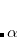-spin NBOs, in the case of an unrestricted SCF calculation. (For ground states, there is typically one strongly-occupied NBO for each electron.) NBO cube files are saved to the plots sub-directory of the job’s scratch directory. One final caveat: to get NBO cube files, the user must specify the AONBO option in the$nbo section, as shown in the following example.

Example 11.250  Generating cube files for the NBOs of the first excited state of.

$rem METHOD CIS BASIS CC-PVTZ CIS_N_ROOTS 1 CIS_TRIPLETS FALSE NBO 2 ! ground- and excited-state NBO MAKE_CUBE_FILES NBOS ! generate NBO cube files... CUBEFILE_STATE 1 ! ...for the first excited state$end

$nbo AONBO$end

$molecule 0 1 O H 1 0.95 H 1 0.95 2 104.5$end

$plots Plot the 5 high-occupancy NBOs, one for each alpha electron 40 -8.0 8.0 40 -8.0 8.0 40 -8.0 8.0 5 0 0 0 1 2 3 4 5$end


## 11.5.5 NCI Plots(11.16)

provides a convenient indicator of noncovalent interactions, which are characterized by large density gradients in regions of space where the density itself is small, leading to very large values of. Q-Chem can generate noncovalent interactions (NCI) plots of the functionin three-dimensional space. To generate these, set the PLOT_REDUCED_DENSITY_GRAD $rem variable to TRUE. (See the nci-c8h14.in input example in$QC/samples directory.)

## 11.5.6 Electrostatic Potentials

Q-Chem can evaluate electrostatic potentials on a grid of points. Electrostatic potential evaluation is controlled by the $rem variable IGDESP, as documented below. IGDESP  Controls evaluation of the electrostatic potential on a grid of points. If enabled, the output is in an ASCII file, plot.esp, in the formatesp for each point. TYPE:  INTEGER DEFAULT:  none no electrostatic potential evaluation OPTIONS:same as the option ’-1’, plus evaluate the ESP of$external_charges$read grid input via the$plots section of the input deckGenerate the ESP values at all nuclear positions +readgrid points in bohr from the ASCII file ESPGrid

RECOMMENDATION:
 None

The following example illustrates the evaluation of electrostatic potentials on a grid, note that IANLTY must also be set to 200.

Example 11.251  A job that evaluates the electrostatic potential for Hon a 1 by 1 by 15 grid, along the bond axis. The output is in an ASCII file called plot.esp, which lists for each grid point,,,, and the electrostatic potential.

$molecule 0 1 H 0.0 0.0 0.35 H 0.0 0.0 -0.35$end

$rem METHOD hf BASIS 6-31g** IANLTY 200 IGDESP -1$end

$plots plot the HOMO and the LUMO on a line 1 0.0 0.0 1 0.0 0.0 15 -3.0 3.0 0 0 0 0 0$end


We can also compute the electrostatic potential for the transition density, which can be used, for example, to compute the Coulomb coupling in excitation energy transfer.

ESP_TRANS
 Controls the calculation of the electrostatic potential of the transition density

TYPE:
 LOGICAL

DEFAULT:
 FALSE

OPTIONS:
 TRUE compute the electrostatic potential of the excited state transition density FALSE compute the electrostatic potential of the excited state electronic density

RECOMMENDATION:
 NONE

The electrostatic potential is a complicated object and it is not uncommon to model it using a simplified representation based on atomic charges. For this purpose it is well known that Mulliken charges perform very poorly. Several definitions of ESP-derived atomic charges have been given in the literature, however, most of them rely on a least-squares fitting of the ESP evaluated on a selection of grid points. Although these grid points are usually chosen so that the ESP is well modeled in the “chemically important” region, it still remains that the calculated charges will change if the molecule is rotated. Recently an efficient rotationally invariant algorithm was proposed that sought to model the ESP not by direct fitting, but by fitting to the multipole moments. By doing so it was found that the fit to the ESP was superior to methods that relied on direct fitting of the ESP. The calculation requires the traceless form of the multipole moments and these are also printed out during the course of the calculations. To request these multipole-derived charges the following \$rem option should be set:

MM_CHARGES
 Requests the calculation of multipole-derived charges (MDCs).

TYPE:
 LOGICAL

DEFAULT:
 FALSE

OPTIONS:
 TRUE Calculates the MDCs and also the traceless form of the multipole moments

RECOMMENDATION:
 Set to TRUE if MDCs or the traceless form of the multipole moments are desired. The calculation does not take long.